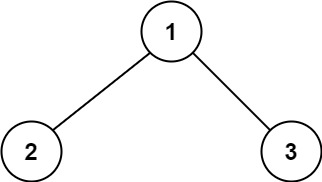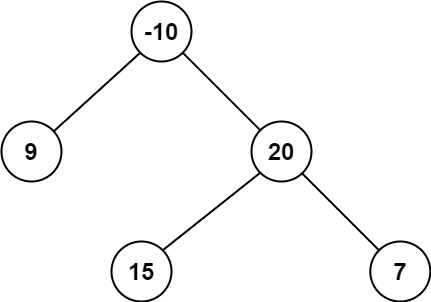# 124.binary-tree-maximum-path-sum

## Statement

• Difficulty: Hard
• Tag: `树` `深度优先搜索` `动态规划` `二叉树```````输入：root = [1,2,3]``````输入：root = [-10,9,20,null,null,15,7]

``````

• 树中节点数目范围是 `[1, 3 * 104]`
• `-1000 <= Node.val <= 1000`

• Link: Binary Tree Maximum Path Sum
• Difficulty: Hard
• Tag: `Tree` `Depth-First Search` `Dynamic Programming` `Binary Tree`

A path in a binary tree is a sequence of nodes where each pair of adjacent nodes in the sequence has an edge connecting them. A node can only appear in the sequence at most once. Note that the path does not need to pass through the root.

The path sum of a path is the sum of the node's values in the path.

Given the `root` of a binary tree, return the maximum path sum of any non-empty path.

Example 1:``````Input: root = [1,2,3]
Output: 6
Explanation: The optimal path is 2 -> 1 -> 3 with a path sum of 2 + 1 + 3 = 6.
``````

Example 2:``````Input: root = [-10,9,20,null,null,15,7]
Output: 42
Explanation: The optimal path is 15 -> 20 -> 7 with a path sum of 15 + 20 + 7 = 42.
``````

Constraints:

• The number of nodes in the tree is in the range `[1, 3 * 104]`.
• `-1000 <= Node.val <= 1000`

## Solution

``````# Definition for a binary tree node.
# class TreeNode:
#     def __init__(self, val=0, left=None, right=None):
#         self.val = val
#         self.left = left
#         self.right = right
from typing import Optional

class Solution:
res = -1000

def dfs(self, root: TreeNode) -> int:
if not root:
return 0

left = self.dfs(root.left)
right = self.dfs(root.right)
val = root.val

self.res = max(self.res, left + right + val,
left + val, right + val, val)
return max(val, left + val, right + val)

def maxPathSum(self, root: Optional[TreeNode]) -> int:
self.dfs(root)
return self.res
``````